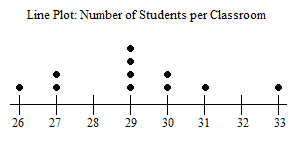# Line Plots

One of the most basic ways to display statistical data is a line plot (or dot plot ). This is used for surveys of small numbers of people, or to show a distribution of data about a small set.

Example:

Suppose you count the number of students in each classroom in your school.

 Class Number of Students Class $1$ $26$ Class $2$ $27$ Class $3$ $29$ Class $4$ $27$ Class $5$ $29$ Class $6$ $31$ Class $7$ $29$ Class $8$ $30$ Class $9$ $30$ Class $10$ $29$ Class $11$ $33$

Since there are only a few classrooms, you can use a line plot to organize your data.

Write the portion of the number line starting with the lowest value up to the highest value in your data set. Then for each classroom, make a mark above the number of students.